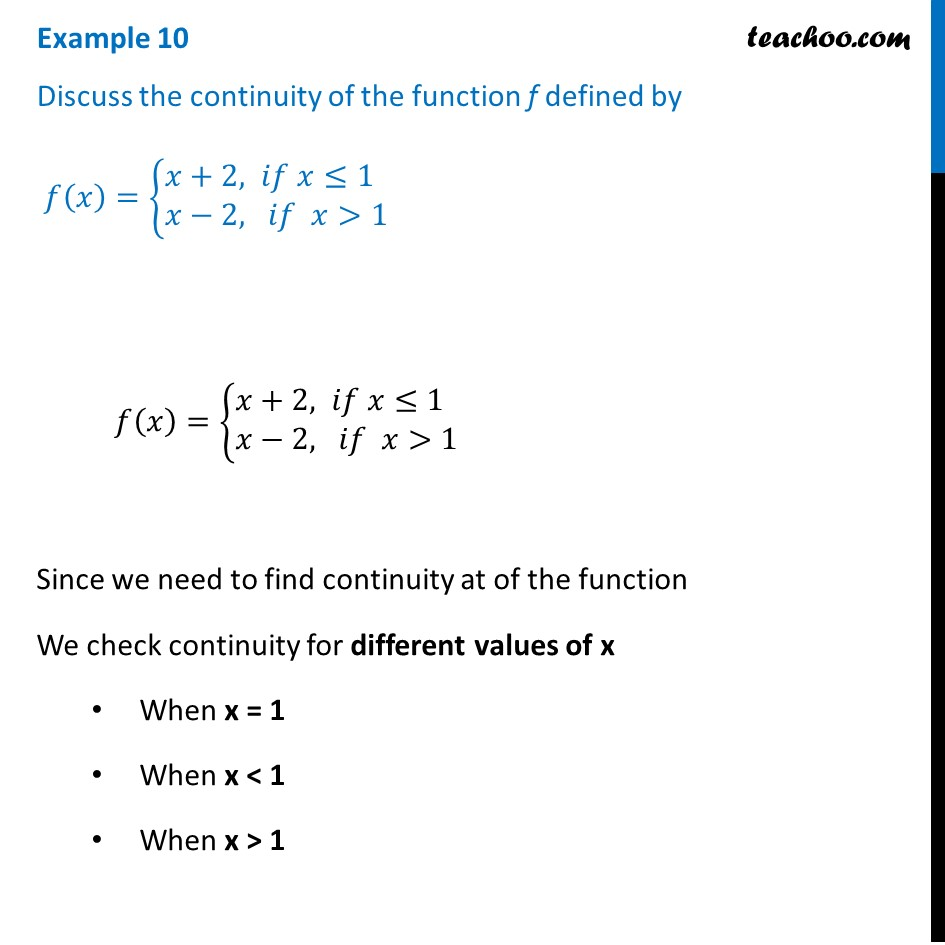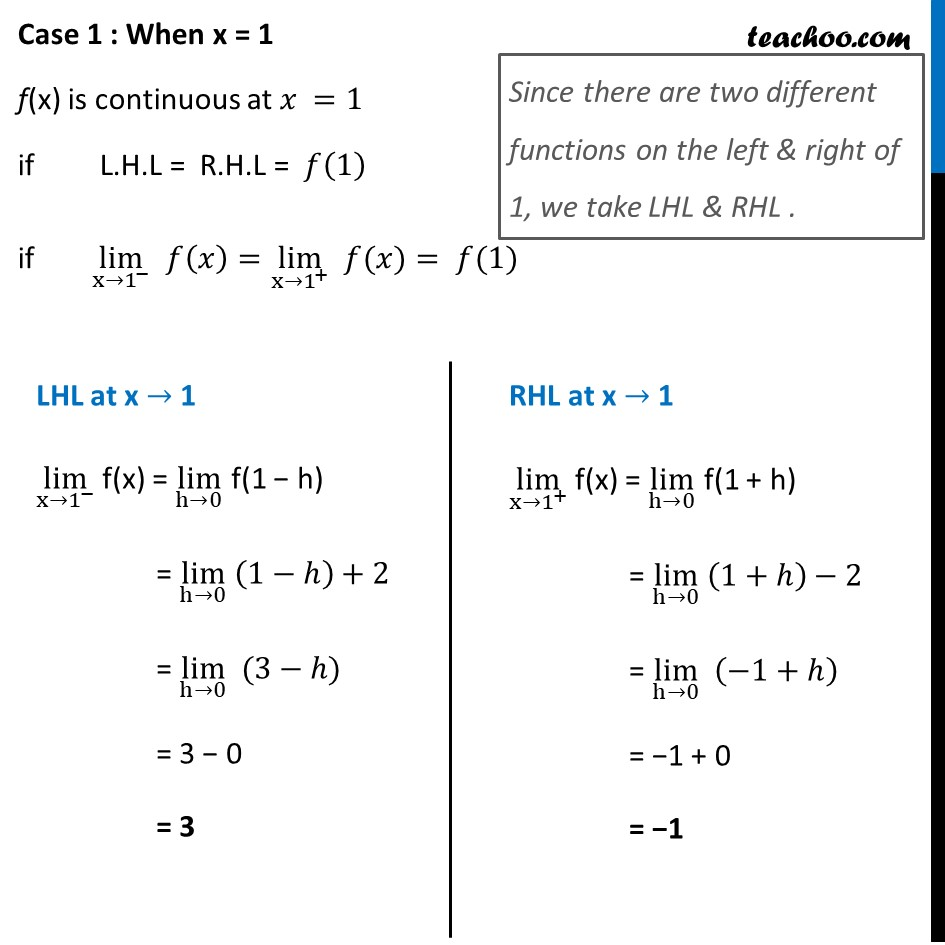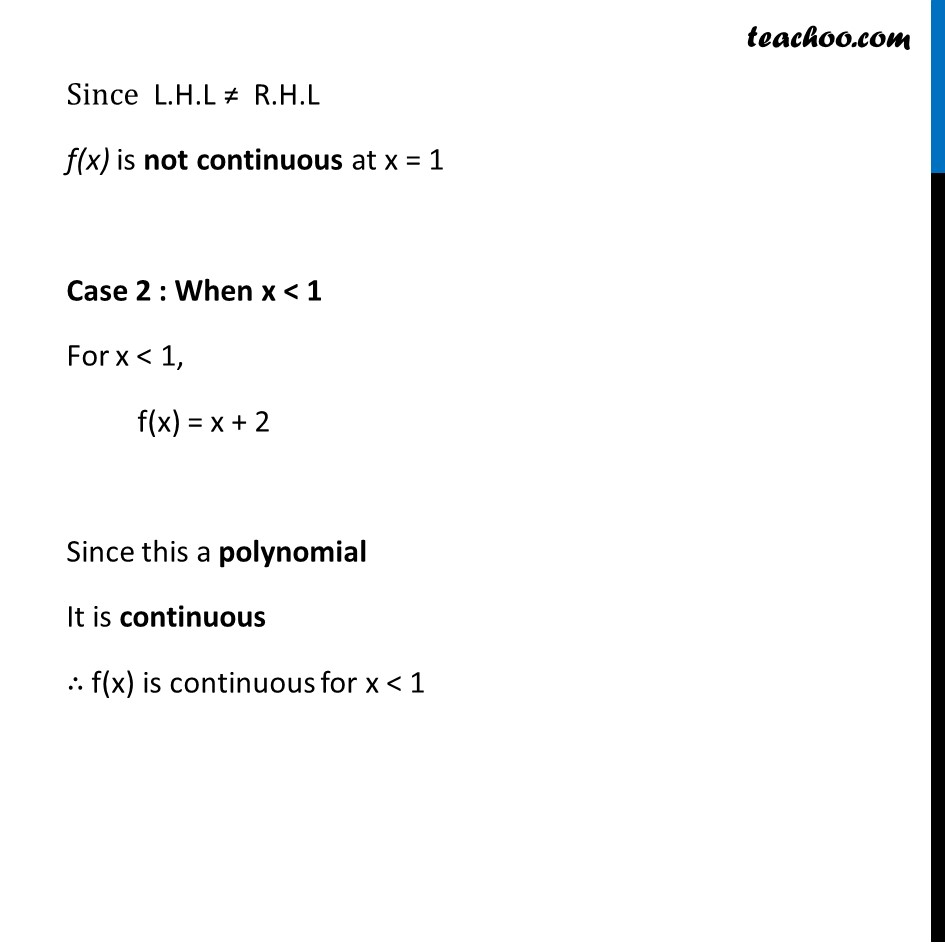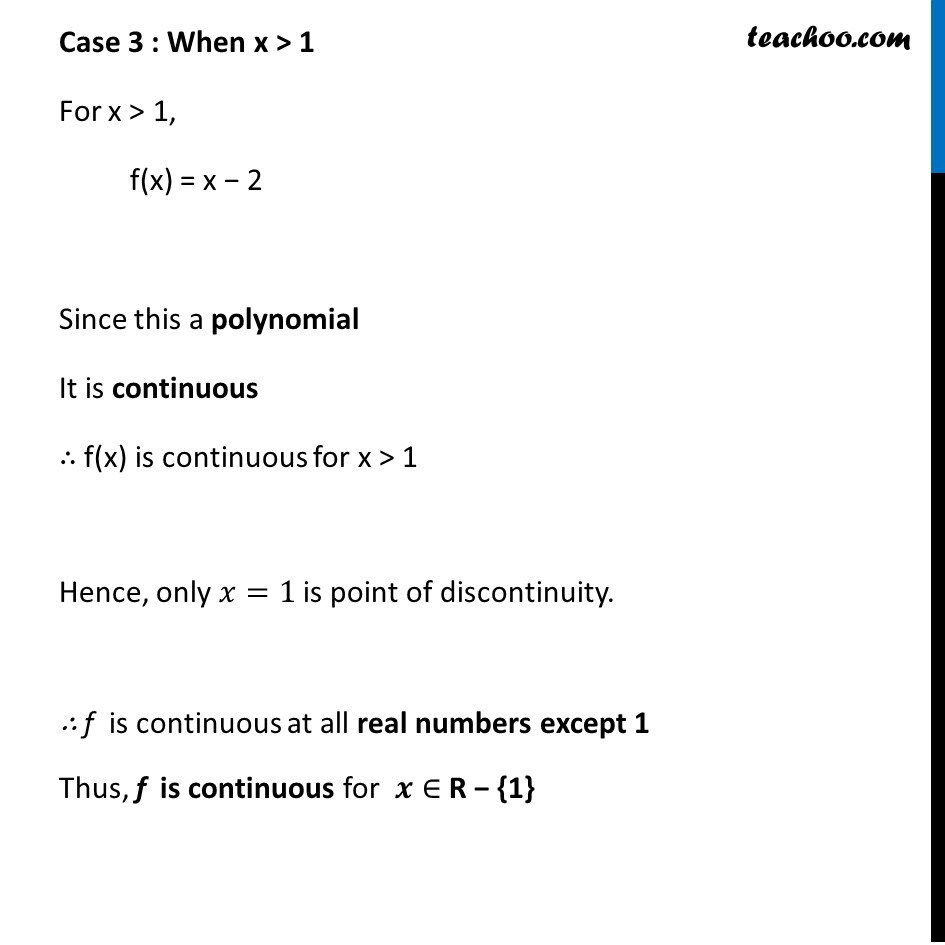Examples

Chapter 5 Class 12 Continuity and Differentiability
Serial order wiseLearn in your speed, with individual attention - Teachoo Maths 1-on-1 Class

### Transcript

Example 10 Discuss the continuity of the function f defined by 𝑓(𝑥)={█(&𝑥+2, 𝑖𝑓 𝑥≤1@&𝑥−2, 𝑖𝑓 𝑥>1)┤ 𝑓(𝑥)={█(&𝑥+2, 𝑖𝑓 𝑥≤1@&𝑥−2, 𝑖𝑓 𝑥>1)┤ Since we need to find continuity at of the function We check continuity for different values of x When x = 1 When x < 1 When x > 1Case 1 : When x = 1 f(x) is continuous at 𝑥 =1 if L.H.L = R.H.L = 𝑓(1) if lim┬(x→1^− ) 𝑓(𝑥)=lim┬(x→1^+ ) " " 𝑓(𝑥)= 𝑓(1) Since there are two different functions on the left & right of 1, we take LHL & RHL . LHL at x → 1 lim┬(x→1^− ) f(x) = lim┬(h→0) f(1 − h) = lim┬(h→0) (1−ℎ)+2 = lim┬(h→0) (3−ℎ) = 3 − 0 = 3 RHL at x → 1 lim┬(x→1^+ ) f(x) = lim┬(h→0) f(1 + h) = lim┬(h→0) (1+ℎ)−2 = lim┬(h→0) (−1+ℎ) = −1 + 0 = −1 Since L.H.L ≠ R.H.L f(x) is not continuous at x = 1 Case 2 : When x < 1 For x < 1, f(x) = x + 2 Since this a polynomial It is continuous ∴ f(x) is continuous for x < 1 Case 3 : When x > 1 For x > 1, f(x) = x − 2 Since this a polynomial It is continuous ∴ f(x) is continuous for x > 1 Hence, only 𝑥=1 is point of discontinuity. ∴ f is continuous at all real numbers except 1 Thus, f is continuous for 𝒙∈ R − {1}